# GPT Transformer Trained onBookCorpus Data

Generate text in English and represent text as a sequence of vectors

Released in 2018, this Generative Pre-Training Transformer (GPT) model is pre-trained in an unsupervised fashion on a large corpus of English text. This model can be further fine-tuned with additional output layers to create highly accurate NLP models for a wide range of tasks. It uses bi-directional causal self-attention, often referred to as a transformer decoder.

Number of models: 2

## Training Set Information

• BookCorpus, a dataset consisting of 11,038 unpublished books from 16 different genres.

## Performance

• The model fine-tuned on various datasets obtains the following accuracy on various natural language inference tasks: 82.1%, 81.4%, 89.9%, 88.3%, 88.1% and 56% accuracy on MNLI-m, MNLI-mm, SNLI, SciTail, QNLI, and RTE datasets respectively.

For question answering and commonsense reasoning, the fine-tuned model obtains the following accuracies: 86.5%, 62.9%, 57.4%, and 59.0% accuracy on Story Cloze, RACE-m, RACE-h, and RACE datasets respectively

## Examples

### Resource retrieval

Get the pre-trained net:

 In:=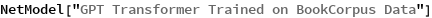Out=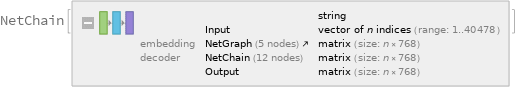### NetModel parameters

This model consists of a family of individual nets, each identified by a specific parameter combination. Inspect the available parameters:

 In:=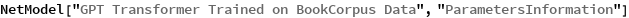Out=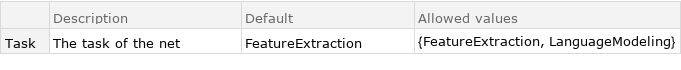Pick a non-default net by specifying the parameters:

 In:=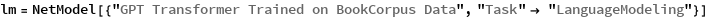Out=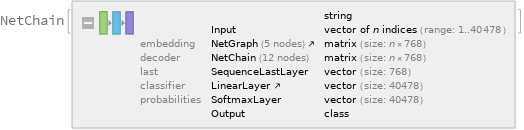Pick a non-default uninitialized net:

 In:=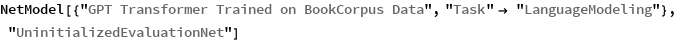Out=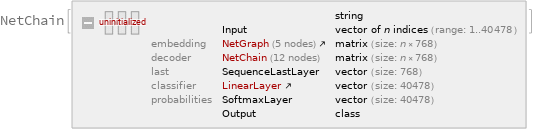### Basic usage

Given a piece of text, the GPT net produces a sequence of feature vectors of size 768, which correspond to the sequence of input words or subwords:

 In:=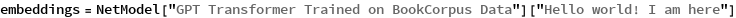Out=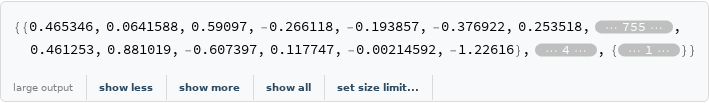Obtain dimensions of the embeddings:

 In:=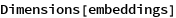Out=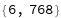Visualize the embeddings:

 In:=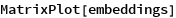Out=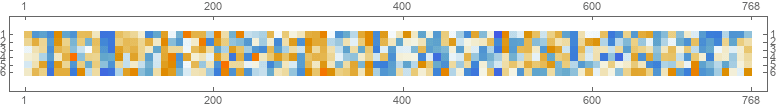### Transformer architecture

The input string is first normalized and then tokenized, or split into words or subwords. This two-step process is accomplished using the NetEncoder "Function":

 In:=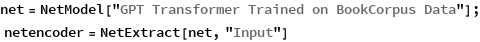Out=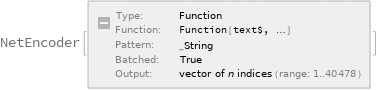The tokenization step is performed using the NetEncoder "BPESubwordTokens" and can be extracted using the following steps:

 In:=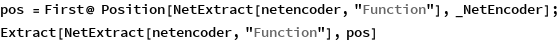The encoder produces an integer index for each subword token that corresponds to the position in the vocabulary:

 In:=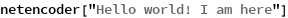Out=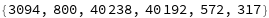Each subword token is also assigned a positional index:

 In:=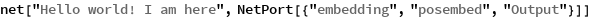Out=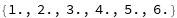A lookup is done to map these indices to numeric vectors of size 768:

 In:=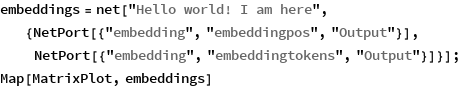Out=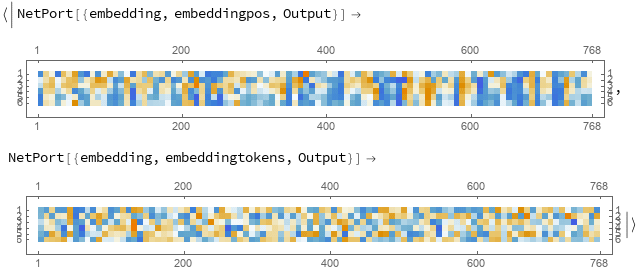For each subword token, these two embeddings are combined by summing elements with ThreadingLayer:

 In:=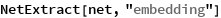Out=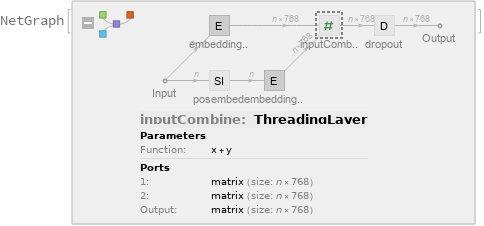The transformer architecture then processes the vectors using 12 structurally identical self-attention blocks stacked in a chain:

 In:=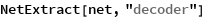Out=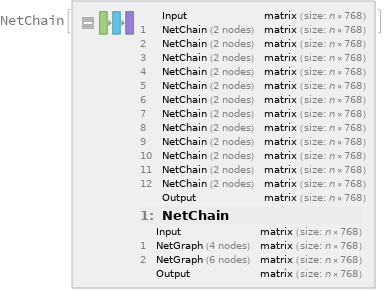The key part of these blocks is the attention module consisting of 12 parallel self-attention transformations, also called “attention heads”:

 In:=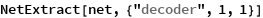Out=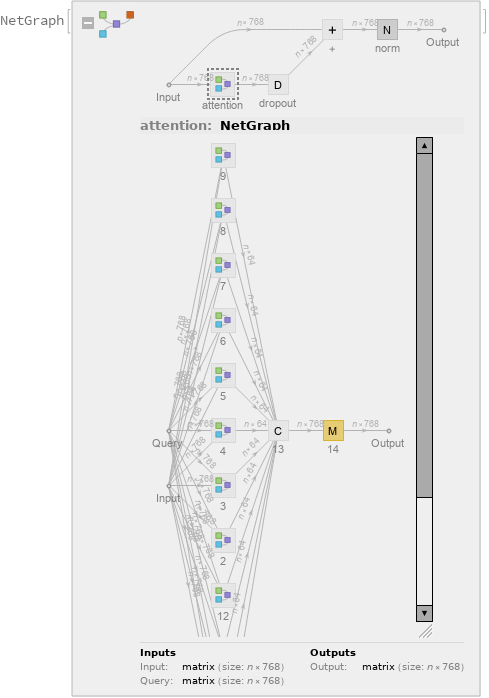Each head uses an AttentionLayer at its core:

 In:=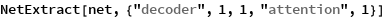Out=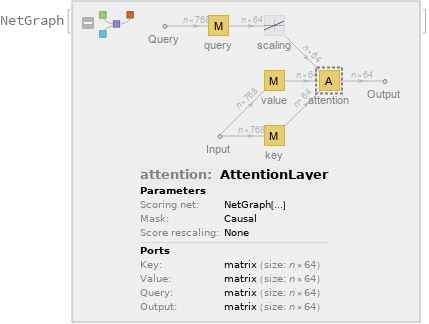Attention is done with causal masking, which means that the embedding of a given subword token depends on the previous subword tokens and not on the subsequent ones. This is a prerequisite to be able to generate text with the language model. The following figures compare causal attention to other forms of connectivity between input tokens: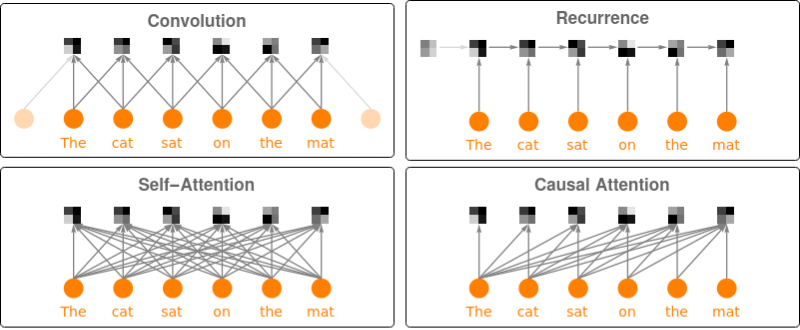### Language modeling: Basic usage

Retrieve the language model by specifying the "Task" parameter:

 In:=Out=Predict the next word in a given sequence:

 In:=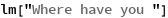Out=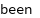Obtain the top 15 probabilities:

 In:=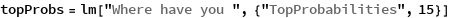Out=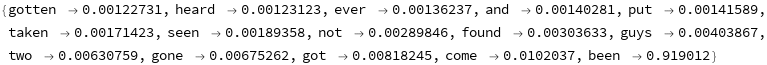Plot the top 15 probabilities:

 In:=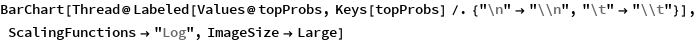Out=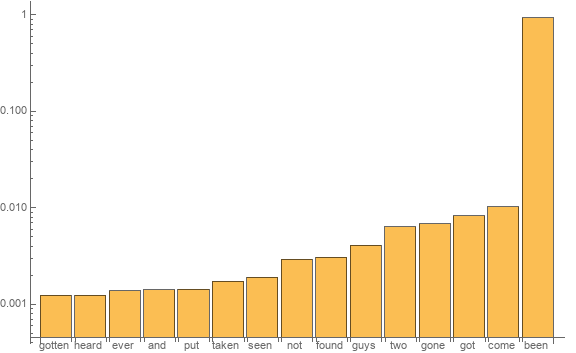### Text generation

Modify the language model so that it accepts the encoded token indices as input and creates the token indices as output:

 In:=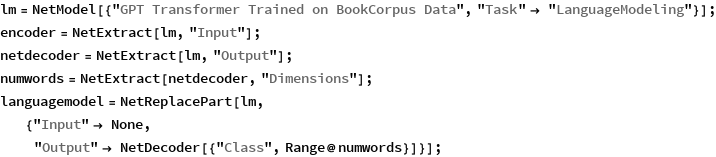Create a new decoder that performs a lookup to find the corresponding string, followed by some text cleaning:

 In:=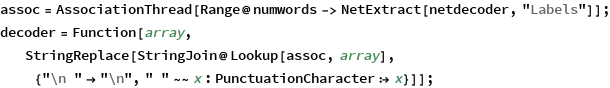Define a function to predict the next token using the modified language model:

 In:=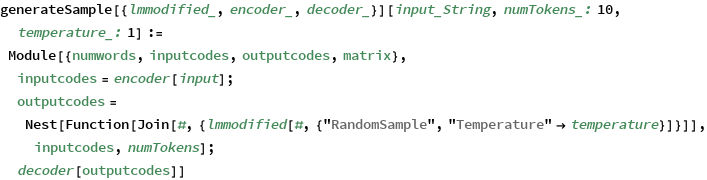Get an input:

 In:=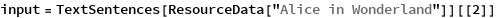Out=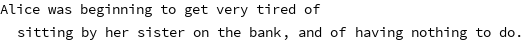Generate the next 20 tokens by using it on the input:

 In:=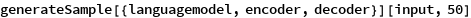Out=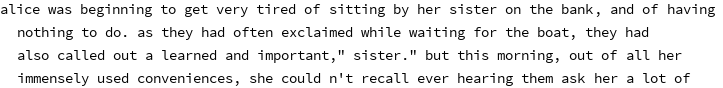The third optional argument is a “temperature” parameter that scales the input to the final softmax. A high temperature flattens the distribution from which tokens are sampled, increasing the probability of extracting less likely tokens:

 In:=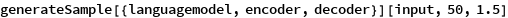Out=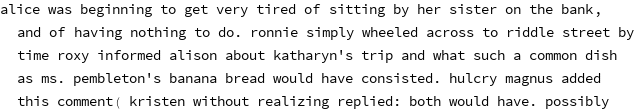Decreasing the temperature sharpens the peaks of the sampling distribution, further decreasing the probability of extracting less likely tokens:

 In:=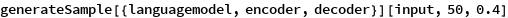Out=Very high temperature settings are equivalent to random sampling:

 In:=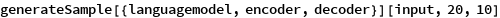Out=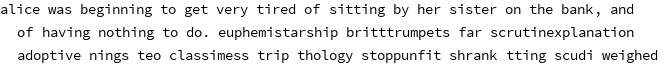Very low temperature settings are equivalent to always picking the character with maximum probability. It is typical for sampling to “get stuck in a loop”:

 In:=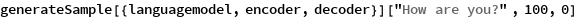Out=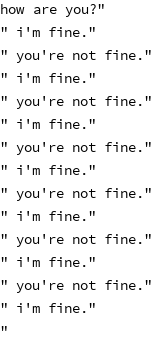### Sentence analogies

Define a sentence embedding that consists of the last subword embedding of GPT (this choice is justified by the fact that GPT is a forward causal model):

 In:=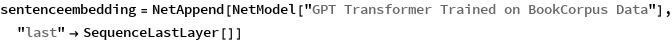Out=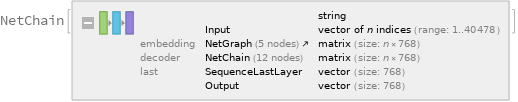Define some sentences in two broad categories for comparison:

 In:=Precompute the embeddings for a list of sentences:

 In:=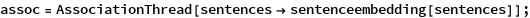Visualize the similarity between the sentences using the net as a feature extractor:

 In:=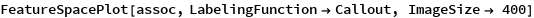Out=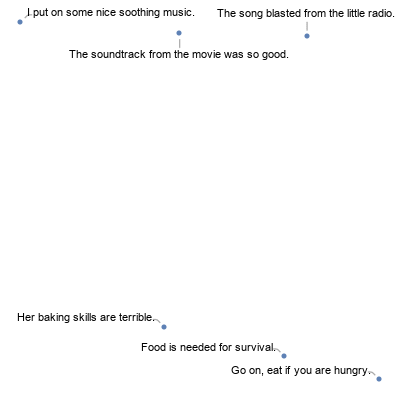### Train a classifier with the subword embeddings

Get a text-processing dataset:

 In:=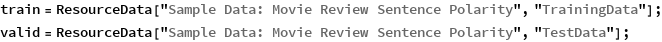View a random sample of the dataset:

 In:=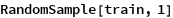Out=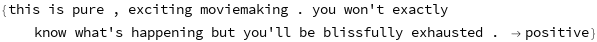Define a sentence embedding that consists of the last subword embedding of GPT (this choice is justified by the fact that GPT is a forward causal model):

 In:=Out=Precompute the GPT vectors for the training and the validation datasets (if available, GPU is highly recommended):

 In:=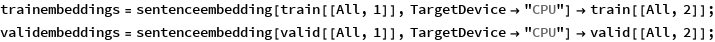Define a simple network for classification:

 In:=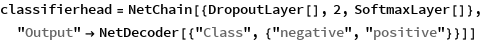Out=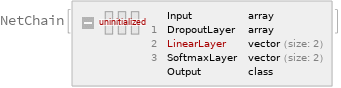Train the network on the precomputed GPT vectors:

 In:=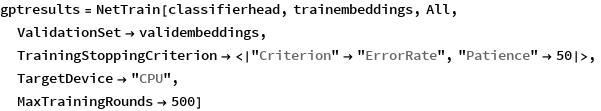Out=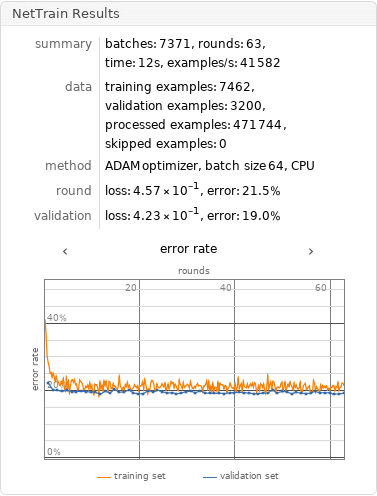Check the classification error rate on the validation data:

 In:=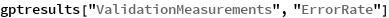Out=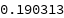Compare the results with the performance of a classifier trained on context-independent word embeddings. Precompute the GloVe vectors for the training and the validation datasets (if available, GPU is recommended):

 In:=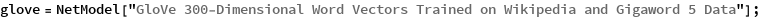In:=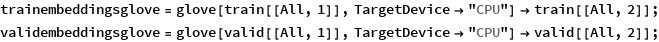Define a simple network for classification, using a max-pooling strategy:

 In:=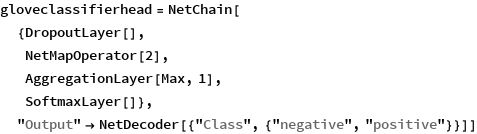Out=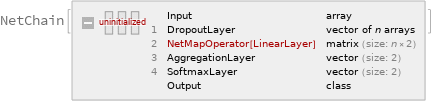Train the classifier on the precomputed GloVe vectors:

 In:=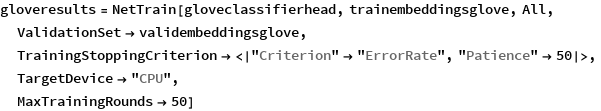Out=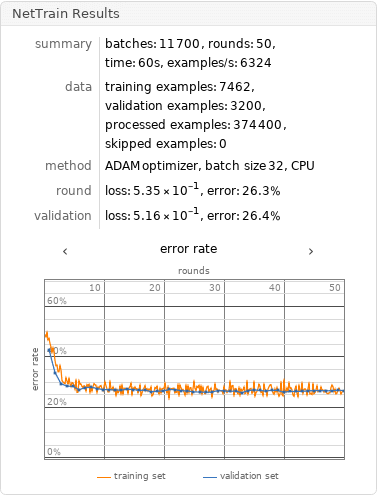Compare the results obtained with GPT and with GloVe:

 In:=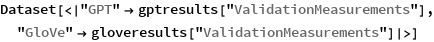Out=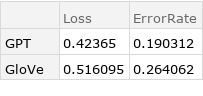### Net information

Inspect the number of parameters of all arrays in the net:

 In:=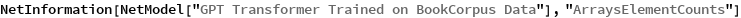Out=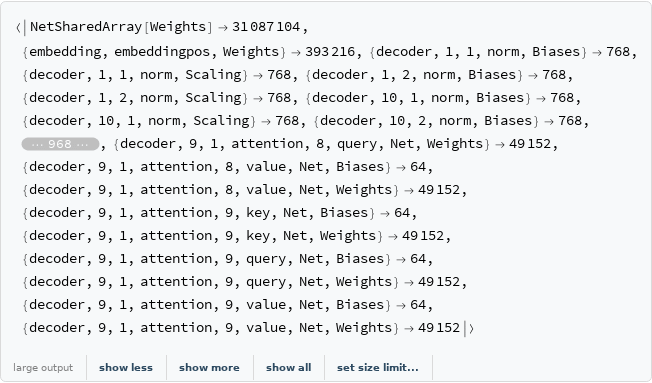Obtain the total number of parameters:

 In:=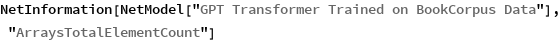Out=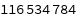Obtain the layer type counts:

 In:=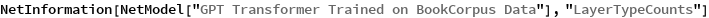Out=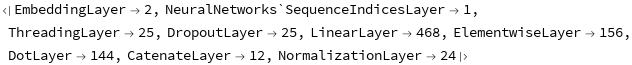Display the summary graphic:

 In:=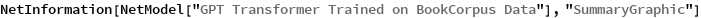Out=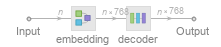### Export to MXNet

Export the net into a format that can be opened in MXNet:

 In:=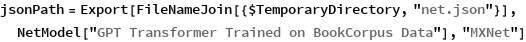Out=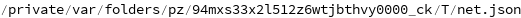Export also creates a net.params file containing parameters:

 In:=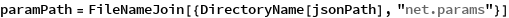Out=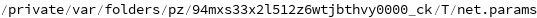Get the size of the parameter file:

 In:=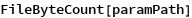Out=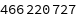## Requirements

Wolfram Language 12.0 (April 2019) or above